Next: Clausius-Clapeyron Equation Up: Multi-Phase Systems Previous: Stability of Single-Phase Substance

# Equilibrium Between Phases

Consider a system that consists of two phases, which we shall denote by 1 and 2. For example, these might be solid and liquid, or liquid and gas. Suppose that the system is in equilibrium with a reservoir at the constant temperature, and the constant pressure, so that the system always has the temperatureand the mean pressure. However, the system can exist in either one of its two phases, or some mixture of the two. Let us begin by finding the conditions that allow the two phases to coexist in equilibrium with one another.

In accordance with the discussion in Section 9.4, the equilibrium condition is that the Gibbs free energy,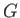, of the system is a minimum: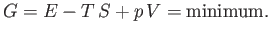(9.59)

Let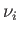be the number of moles of phase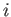present in the system, and let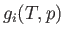be the Gibbs free energy per mole of phaseat the temperatureand the pressure. It follows that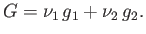(9.60)

Furthermore, the conservation of matter implies that the total number of moles,, of the substance remains constant: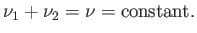(9.61)

Thus, we can take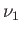as the one independent parameter that is free to vary. In equilibrium, Equation (9.59) requires thatbe stationary for small variations in. In other words,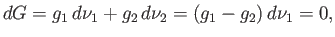(9.62)

because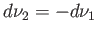, as a consequence of Equation (9.61). Hence, a necessary condition for equilibrium between the two phases is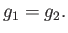(9.63)

Clearly, when this condition is satisfied then the transfer of a mole of substance from one phase to another does not change the overall Gibbs free energy,, of the system. Hence,is stationary, as required. Incidentally, the condition thatis a minimum (rather than a maximum) is easily shown to reduce to the requirement that the heat capacities and isothermal compressibilities of both phases be positive, so that each phase is stable to temperature and volume fluctuations.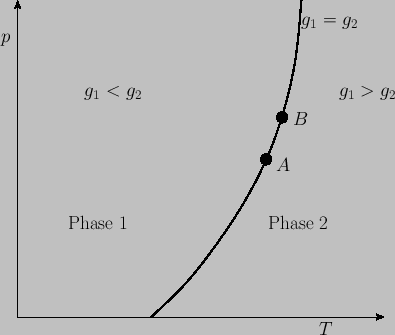Now, for a given temperature and pressure,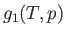is a well-defined function characteristic of phase 1. Likewise,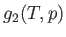is a well-defined function characteristic of phase 2. Ifandare such that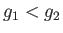then the minimum value ofin Equation (9.59) is achieved when allmoles of the substance transform into phase 1, so that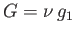. In this case, phase 1 is the stable one. On the other hand, if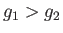then the minimum value ofis achieved when allmoles of the substance transform into phase 2, so that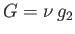. In this case, phase 2 is the stable one. Finally, if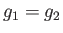then the condition (9.59) is automatically satisfied, and any amount,, of phase 1 can coexist with the remaining amount,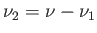, of phase 2. The locus of the points in the-plane wherethen represents a phase-equilibrium line along which the two phases can coexist in equilibrium. This line divides the-plane into two regions. The first corresponds to, so that phase 1 is stable. The second corresponds to, so that phase 2 is stable. See Figure 9.1.Next: Clausius-Clapeyron Equation Up: Multi-Phase Systems Previous: Stability of Single-Phase Substance
Richard Fitzpatrick 2016-01-25